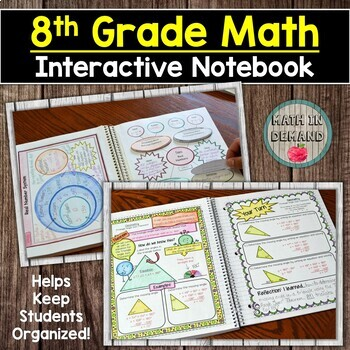### 8th Grade Math Interactive Notebook with Guided Notes and Examples

This is a whole years worth of an interactive notebook that is very organized and engaging. CHECK OUT THE PREVIEW TO SEE HOW AMAZING THIS NOTEBOOK IS!The notebook has 5 units (6 including notebook starter). The units include:

Unit 0 - Notebook Starter

Unit 1 - The Number System

Unit 2 - Expressions & Equations

Unit 3 - Functions

Unit 4 - Geometry

Unit 5 - Statistics & ProbabilityA breakdown of each unit is below:

Unit 0 - Notebook Starter

-Notebook Cover for Students

-Classroom Rules

-Notebook Expectations

-Math Reference Sheet

-Reflection Page

-My Goals: Setting Goals

-My Goals: A Look Back

Unit 1 - The Number System

✅ The Real Number System

✅ Rationals vs Irrationals

✅ Converting a Fraction into a Repeating Decimal

✅ Converting a Repeating Decimal into a Fraction

✅ Square Roots and Cube Roots

✅ Approximating Non-Perfect Squares

✅ Non-Perfect Squares on the Number Line

✅ Comparing Irrational Numbers

✅ Exponent Properties

✅ Intro to Scientific Notation

✅ Adding and Subtracting with Scientific Notation

✅ Multiplying and Dividing with Scientific Notation

Unit 2 - Expressions & Equations

✅ Unit Rate on a Graph

✅ Solving Equations with Variables on Both Sides

✅ Equations with Parentheses

✅ Number of Solutions to Linear Equations

✅ X and Y intercepts from a Table and Graph

✅ X and Y intercepts from an Equation

✅ Graphing using Intercepts

✅ Types of Slope

✅ Determining Slope Given a Graph

✅ Determining Slope Given a Table or Two Points

✅ Slope-Intercept Form

✅ Writing Linear Equations from a Graph

✅ Writing Linear Equations Given Two Points

✅ Standard Form to Slope-Intercept Form

✅ Types of Solutions from a Systems of Equations

✅ Solving Systems of Equations by Graphing

✅ Solving Systems of Equations by Substitution

✅ Solving Systems of Equations by Elimination

✅ Solving Systems of Equations Word Problems

Unit 3 - Functions

✅ Intro to Functions

✅ Function Notation and Evaluating a Function

✅ Linear vs Non-Linear

✅ Writing Linear Functions from a Table

✅ Comparing Functions (Graph, Table, Equation, and Verbal Description)

Unit 4 - Geometry

✅ Intro to Transformations

✅ Rigid Transformations: Reflections, Rotations, & Translations Rules

✅ Dilations

✅ Sequence of Transformations

✅ Similarity

✅ Congruence

✅ Identifying Angle Relationships

✅ Solving with Angle Relationships

✅ Triangle Angle Sum Theorem

✅ Triangle Exterior Angle Theorem

✅ Proving the Pythagorean Theorem

✅ Applying the Pythagorean Theorem

✅ Pythagorean Theorem in Coordinate System

✅ Volume: Cone, Cylinder, and Sphere

Unit 5 - Statistics & Probability

✅ Intro to Scatter Plots

✅ Constructing Scatter Plots

✅ Line of Best Fit

✅ Two Way TablesThis notebook is absolutely amazing! It includes 2 pages for each concept. The first page is more aimed towards direct instruction / notes. The second page is "Your Turn". Students will apply their newly acquired knowledge to solve problems. After solving problems, they will rate themselves on how well they understand the concepts and write a reflection.The interactive notebook does not include my wheel foldables.

I also have a 6th grade and 7th grade math interactive notebook. I have included a link at the bottom of the description to see a sample of this notebook. In addition, I have included the links to the 6th and 7th grade notebook.

This notebook is a zip file containing pdf files.

Related Products

Connect with Me!

This resource includes a limited use license from Math in Demand. You may only use the resource for personal classroom use. This purchase does not allow you to transfer my resource to another teacher, school, or etc. You must purchase an additional license at a discounted cost.

Questions? Please email me at mathindemand@hotmail.com or click the Q&A tab.